Study Guide

# Basic Probability

## Basic Probability

### Dice and Probability

If we roll a single, standard die, what is the probability of rolling a 4?

If we roll a single, standard die (no, not die as in keel over, but die as in only one of two dice), there are six different numbers we could possibly roll: 1, 2, 3, 4, 5, or 6, and only one of these numbers is a 4.

Since rolling a 4 is the favorable outcome, and there’s only one 4, the numerator of the probability is 1. There are six possible outcomes, so the denominator is 6. The probability of rolling a 4 is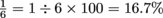.

Now, let’s say we roll a non-standard die that has the following numbers on its six sides: 1, 1, 2, 2, 3, 3. What is the probability of rolling a 3?

With this funky die the number 3 shows up twice, so there are 2 favorable outcomes, making the numerator 2. There are still six possible outcomes so the denominator is still 6, making the probability. We can, and should, simplify probability fractions when possible so the answer to this probability problem is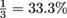.

Now for two dice. What is the probability that the sum of two six-sided dice will be greater than 10?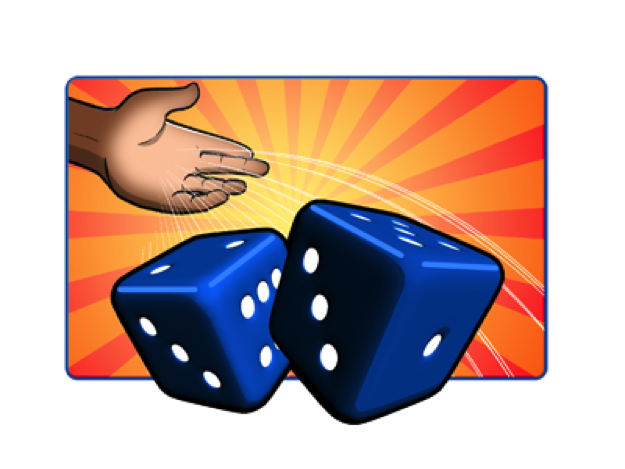First, we need to look at all of the possible sums we can get from rolling two dice. Each die has these possible outcomes: 1, 2, 3, 4, 5, and 6. We can make a table to represent the combinations.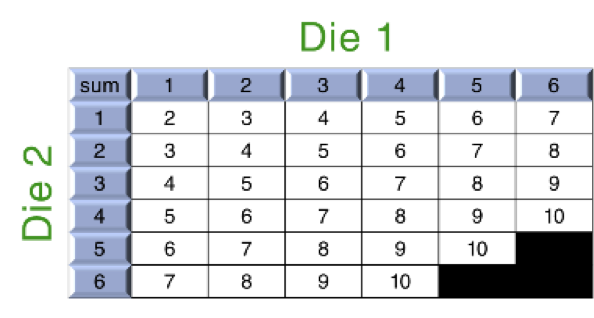As you can see there are 36 possible combinations, 3 of which are greater than 10, and these are shaded. So, we have 36 possible outcomes and 3 favorable ones: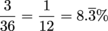### Playing Cards and Probability

What is the probability of drawing a 10 of diamonds randomly from a standard deck of 52 cards?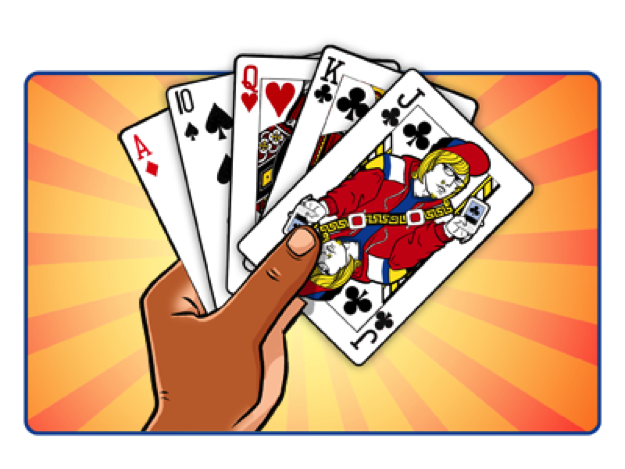First, it's important to know what is in a deck of cards.

52 cards (not including Jokers)
26 red and 26 black
4 suits: diamonds, clubs, hearts, and spades
diamonds and hearts are red
13 cards in each suit: A, 2, 3, 4, 5, 6, 7, 8, 9, 10, J, Q, K
3 face cards in each suit: Jack, Queen, King

Since there are 52 cards and only one of them is a 10 of diamonds, the probability is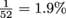. Pretty slim chance, huh?

What about pulling any diamond card? The probability of pulling a diamond card would be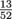since there are 13 cards in the suit of diamonds, and 52 cards in the total deck of cards. Simplifying the fraction, we get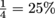. So, there’s a much better chance of pulling a diamond card than pulling a 10 of diamonds.

What is the probability of drawing an ace from a standard deck of 52 cards? The outcome we want is an ace, and there are 4 aces, so the probability is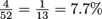.

### Impossible and Certain Events

Okay, one last card trick. What is the probability of drawing a Joker from a standard deck of 52 cards that has no Jokers? Some things are impossible to do and, assuming there are no tricks up our sleeve, this is one of them. But it is possible to calculate the probability. There are 0 Jokers in the deck of 52 cards, so the probability is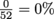. Any event that is impossible has a probability of 0.

Some things are impossible not to do. Hate those double negatives? Let’s say it another way; some things are absolutely certain. Here’s an example. What is the probability of picking either a red or a black card from a standard deck of 52 cards? Well, all the cards are either red or black, all 52 of them. So the probability is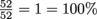.

Let’s say you have a bag of 8 red marbles. What is the probability of reaching in, with your eyes closed, and pulling out a red marble? What is the probability of pulling out a blue marble? Because there are only red marbles, you’re 100% likely to pull out a red marble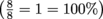. Your probability of pulling out a blue marble is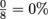. Definitely don’t count on that outcome.